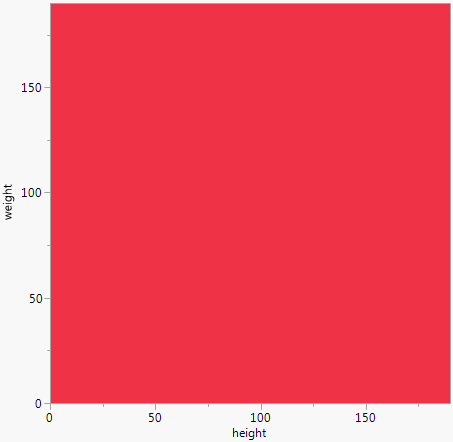Scripting Guide > Scripting Graphs > Create New Graphs from Scratch and Customize Them
Publication date: 04/12/2021

# Create New Graphs from Scratch and Customize Them

Graphics scripts are set up inside the Graph Box() command within a New Window() command.

`New Window("title", <arguments>, Graph Box( named arguments,..., script));`

The following arguments are named arguments for Graph Box():

`Frame Size( horizontal, vertical ), // size in pixels`
`X Scale( xmin, xmax ), Y Scale( ymin, ymax ), // range of x and y axes`
`X Name( "x" ), Y Name( "y" ), // names for x and y axes`
`Suppress Axes // omit the axes`

For example, Graph Box() accepts named arguments as shown in the following example, which creates a graph with a red background.

`win = New Window( "Named arguments",`
`	Graph Box(`
`		Frame Size( 300, 300 ), // construct the graph box`
`		X Scale( 0, 190 ), // set the x-axis range`
`		Y Scale( 0, 190), // set the y-axis range`
`		X Name( "height" ),`
`		Y Name( "weight" ),`
`		<<Background Color( "Red" ) // set the background color`
`	)`
`);`

Figure 12.7 Creating a GraphAlternatively, you can use the send << operator to send commands to the Graph Box instead of using the named arguments. This example also creates a graph with a red background.

`win = New Window( "Messages",`
`	Graph Box(`
`		<<Frame Size( 300, 300 ),`
`		<<XAxis( 0, 190 ),`
`		<<YAxis( 0, 190 ),`
`		<<Background Color( "Red" )`
`	)`
`);`
Want more information? Have questions? Get answers in the JMP User Community (community.jmp.com).